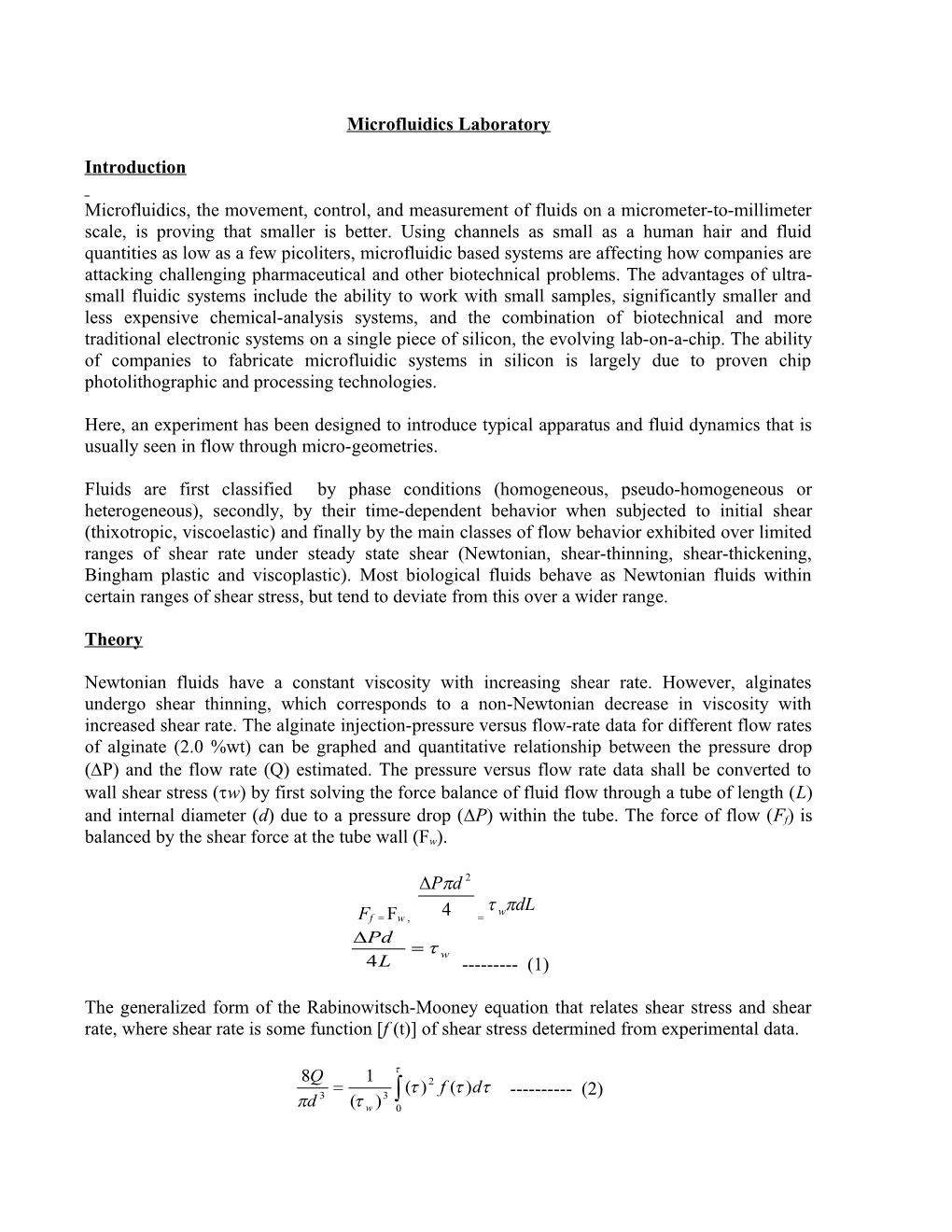# Microfluidics LaboratoryMicrofluidics Laboratory

Introduction

Microfluidics, the movement, control, and measurement of fluids on a micrometer-to-millimeter scale, is proving that smaller is better. Using channels as small as a human hair and fluid quantities as low as a few picoliters, microfluidic based systems are affecting how companies are attacking challenging pharmaceutical and other biotechnical problems. The advantages of ultra-small fluidic systems include the ability to work with small samples, significantly smaller and less expensive chemical-analysis systems, and the combination of biotechnical and more traditional electronic systems on a single piece of silicon, the evolving lab-on-a-chip. The ability of companies to fabricate microfluidic systems in silicon is largely due to proven chip photolithographic and processing technologies.

Here, an experiment has been designed to introduce typical apparatus and fluid dynamics that is usually seen in flow through micro-geometries.

Fluids are first classified by phase conditions (homogeneous, pseudo-homogeneous or heterogeneous), secondly, by their time-dependent behavior when subjected to initial shear (thixotropic, viscoelastic) and finally by the main classes of flow behavior exhibited over limited ranges of shear rate under steady state shear (Newtonian, shear-thinning, shear-thickening, Bingham plastic and viscoplastic). Most biological fluids behave as Newtonian fluids within certain ranges of shear stress, but tend to deviate from this over a wider range.

Theory

Newtonian fluids have a constant viscosity with increasing shear rate. However, alginates undergo shear thinning, which corresponds to a non-Newtonian decrease in viscosity with increased shear rate. The alginate injection-pressure versus flow-rate data for different flow rates of alginate (2.0 %wt) can be graphed and quantitative relationship between the pressure drop (P) and the flow rate (Q) estimated. The pressure versus flow rate data shall be converted to wall shear stress (w) by first solving the force balance of fluid flow through a tube of length (L) and internal diameter (d) due to a pressure drop (P) within the tube. The force of flow (Ff) is balanced by the shear force at the tube wall (Fw).

Ff = Fw , =

------(1)

The generalized form of the Rabinowitsch-Mooney equation that relates shear stress and shear rate, where shear rate is some function [f (t)] of shear stress determined from experimental data.

------(2)

As the results will show, experimental data best fit a power-law trend indicating that alginate is a non-Newtonian fluid. Therefore, the power-law equation was used as the function relating shear rate to shear stress,

------(3)

where K is the scaling constant. To calculate shear rate at the wall (), the generalized Rabinowitsch-Mooney equation (2) needs to be solved using the power law relationship. The generalized power-law equation for wall shear rate becomes,

= ------(4)

Equation 4 essentially is the Newtonian relation of shear rate to flow, with a power-law coefficient to adjust for the shear-thinning effect. A shear-thinning fluid has an n < 1 and a Newtonian fluid has an n = 1. To estimate the power law coefficient, the power-law equation() was solved for n.

Note: You need not calculate shear stress or the shear rate. Since shear stress is proportional to the P(from (1)) and the shear rate is proportional to Q(from (4)), it suffices to plot a relationship between P and Q to obtain the value of n.

Materials / Instruments needed

Harvard PHD 2000 Infusion/Withdraw Pump

Pressure transducer

Syringes

Needles

Capillaries

Alginate solution (2% wt.)

Viscometer

Stop Clock

Oscilloscope

ETH-250 Bridge/Bioamplifier amplifier

Estimating the calibration curve of the bridge amplifier

Amplifier settings during calibration

Mode: Bridge Filter: 4 Hz Gain: 100

The calibration curve will be provided to you.

## Offset Null

Before taking the readings, one has to null the offset voltage reading during no flow condition. Connect the pressure transducer to the input of the amplifier. The offset can be nulled using the input offset adjustment knob in the amplifier.

Experimental Procedure

The pressure transducer is fitted to the syringe filled with alginate solution using a T- valve. The third end of the valve is fitted with the needle. The needle is glued using epoxy resin to the capillary through which the alginate solution flows. This setup is now mounted onto the infusion pump (see the figures below).

The pressure transducer output is connected to the amplifier. The amplifier output is connected to the scope.

Different mass flow rates are set in the pump and the steady state pressure value is noted from the scope. In our case, the range of mass flow rate is between 0.1 and 1.0 ml/min for water and between 0.05 and 0.5 ml/min for alginate. You may have to wait for about 5-10 minutes for the voltage readout to settle down in the case of alginate.

Convert the output reading from the scope into pressure value. Plot the mass flow rate against the pressure.Obtain the power law relationship between the pressure drop and flow rate. Include a report with a brief introduction, methods, complete data analysis (graphs, tables, hand calc etc.) and detailed discussion section.